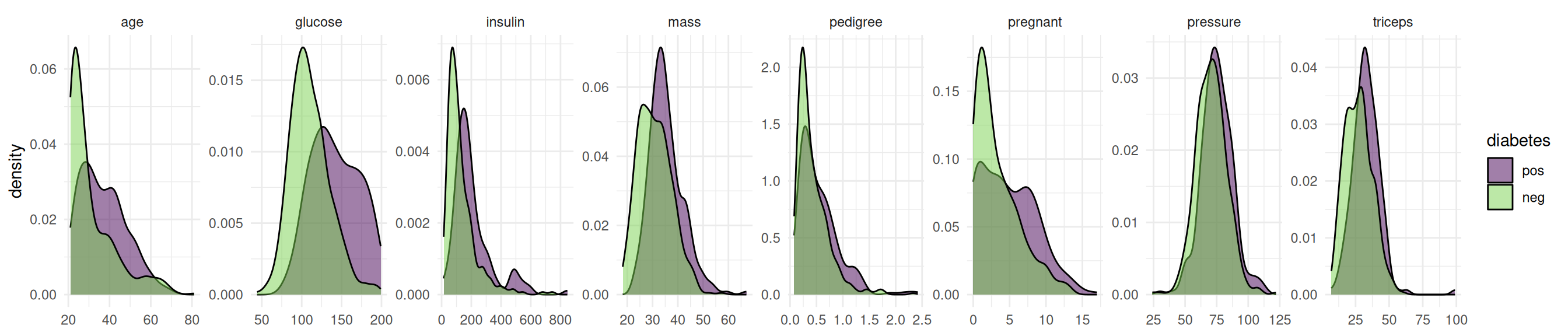# Shadow Variable Search on the Pima Indian Diabetes Data Set

Run a feature selection with permutated features.

Authors
Published

February 1, 2023

# Scope

Feature selection is the process of finding an optimal set of features to improve the performance, interpretability and robustness of machine learning algorithms. In this article, we introduce the Shadow Variable Search algorithm which is a wrapper method for feature selection. Wrapper methods iteratively add features to the model that optimize a performance measure. As an example, we will search for the optimal set of features for a support vector machine on the Pima Indian Diabetes data set. We assume that you are already familiar with the basic building blocks of the mlr3 ecosystem. If you are new to feature selection, we recommend reading the feature selection chapter of the mlr3book first. Some knowledge about mlr3pipelines is beneficial but not necessary to understand the example.

The objective of the Pima Indian Diabetes data set is to predict whether a person has diabetes or not. The data set includes 768 patients with 8 measurements (see Figure 1).

task = tsk("pima")
Code
library(ggplot2)
library(data.table)

data = melt(as.data.table(task), id.vars = task$target_names, measure.vars = task$feature_names)

ggplot(data, aes(x = value, fill = diabetes)) +
geom_density(alpha = 0.5) +
facet_wrap(~ variable, ncol = 8, scales = "free") +
scale_fill_viridis_d(end = 0.8) +
theme_minimal() +
theme(axis.title.x = element_blank())Figure 1: Distribution of the features in the Pima Indian Diabetes data set.

The data set contains missing values.

task$missings() diabetes age glucose insulin mass pedigree pregnant pressure triceps 0 0 5 374 11 0 0 35 227  Support vector machines cannot handle missing values. We impute the missing values with the histogram imputation method. learner = po("imputehist") %>>% lrn("classif.svm", predict_type = "prob") # Feature Selection Now we define the feature selection problem by using the fsi() function that constructs an FSelectInstanceSingleCrit. In addition to the task and learner, we have to select a resampling strategy and performance measure to determine how the performance of a feature subset is evaluated. We pass the "none" terminator because the shadow variable search algorithm terminates by itself. instance = fsi( task = task, learner = learner, resampling = rsmp("cv", folds = 3), measures = msr("classif.auc"), terminator = trm("none") ) We are now ready to start the shadow variable search. To do this, we simply pass the instance to the $optimize() method of the optimizer.

optimizer$optimize(instance)  age glucose insulin mass pedigree pregnant pressure triceps features classif.auc 1: TRUE TRUE FALSE TRUE TRUE FALSE FALSE FALSE age,glucose,mass,pedigree 0.835165 The optimizer returns the best feature set and the corresponding estimated performance. Figure 2 shows the optimization path of the feature selection. The feature glucose was selected first and in the following iterations age, mass and pedigree. Then a shadow variable was selected and the feature selection was terminated. Code library(data.table) library(ggplot2) library(mlr3misc) library(viridisLite) data = as.data.table(instance$archive)[order(-classif.auc), head(.SD, 1), by = batch_nr][order(batch_nr)]
data[, features := map_chr(features, str_collapse)]
data[, batch_nr := as.character(batch_nr)]

ggplot(data, aes(x = batch_nr, y = classif.auc)) +
geom_bar(
stat = "identity",
width = 0.5,
fill = viridis(1, begin = 0.5),
alpha = 0.8) +
geom_text(
data = data,
mapping = aes(x = batch_nr, y = 0, label = features),
hjust = 0,
nudge_y = 0.05,
color = "white",
size = 5
) +
coord_flip() +
xlab("Iteration") +
theme_minimal()

The archive contains all evaluated feature sets. We can see that each feature has a corresponding shadow variable. We only show the variables age, glucose and insulin and their shadow variables here.

as.data.table(instance$archive)[, .(age, glucose, insulin, permuted__age, permuted__glucose, permuted__insulin, classif.auc)]  age glucose insulin permuted__age permuted__glucose permuted__insulin classif.auc 1: TRUE FALSE FALSE FALSE FALSE FALSE 0.6437052 2: FALSE TRUE FALSE FALSE FALSE FALSE 0.7598155 3: FALSE FALSE TRUE FALSE FALSE FALSE 0.4900280 4: FALSE FALSE FALSE FALSE FALSE FALSE 0.6424026 5: FALSE FALSE FALSE FALSE FALSE FALSE 0.5690107 --- 54: TRUE TRUE FALSE FALSE FALSE FALSE 0.8266713 55: TRUE TRUE FALSE FALSE FALSE FALSE 0.8063568 56: TRUE TRUE FALSE FALSE FALSE FALSE 0.8244232 57: TRUE TRUE FALSE FALSE FALSE FALSE 0.8234605 58: TRUE TRUE FALSE FALSE FALSE FALSE 0.8164784 # Final Model The learner we use to make predictions on new data is called the final model. The final model is trained with the optimal feature set on the full data set. We subset the task to the optimal feature set and train the learner. task$select(instance$result_feature_set) learner$train(task)

The trained model can now be used to predict new, external data.

# Conclusion

The shadow variable search is a fast feature selection method that is easy to use. More information on the theoretical background can be found in Wu, Boos, and Stefanski (2007) and Thomas et al. (2017). If you want to know more about feature selection in general, we recommend having a look at our book.

## References

Thomas, Janek, Tobias Hepp, Andreas Mayr, and Bernd Bischl. 2017. “Probing for Sparse and Fast Variable Selection with Model-Based Boosting.” Computational and Mathematical Methods in Medicine 2017 (July): e1421409. https://doi.org/10.1155/2017/1421409.
Wu, Yujun, Dennis D Boos, and Leonard A Stefanski. 2007. “Controlling Variable Selection by the Addition of Pseudovariables.” Journal of the American Statistical Association 102 (477): 235–43. https://doi.org/10.1198/016214506000000843.